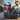# Prime Factors Kata in Scala

September 20, 2012

I like running through the Prime Factors Kata when learning a new language to help uncover the language’s power. I’ve done this before in Clojure and now it’s Scala’s turn. Scala is interesting in that it attempts to merge the best aspects of both object-oriented and functional languages.

The steps I took follow. Each is separated by a horizontal line with changes from the previous iteration in red. The factors of four caused modifications to the algorithm that allowed the next two tests to pass without further changes. It was test-driven using ScalaTest.

`.css-1baulvz{display:inline-block;}test("Factor of one is empty list") {  assert(List[Int]() === primeFactors(1))}`
`def primeFactors(n: Int) = {  List[Int]()}`

`test("Factor of two is two") {  assert(List(2) === primeFactors(2))}`
`def primeFactors(n: Int) = {  if (n > 1) {    List(2)  } else {    List[Int]()  }}`

`test("Factor of three is three") {  assert(List(3) === primeFactors(3))}`
`def primeFactors(n: Int) = {  if (n > 1) {    List(n)  } else {    List[Int]()  }}`

`test("Factors of four are two and two") {  assert(List(2, 2) === primeFactors(4))}`
`def primeFactors(n: Int) = {  def primeFactors(n: Int, candidate: Int): List[Int] =    if (n <= 1) List[Int]()    else if (n % candidate == 0) candidate :: primeFactors(n / candidate, candidate)    else List(n)  primeFactors(n, 2)}`

`test("Factors of six are two and three") {  assert(List(2, 3) === primeFactors(6))}`

`test("Factors of eight are two, two, and two") {  assert(List(2, 2, 2) === primeFactors(8))}`

`test("Factors of nine are three and three") {  assert(List(3, 3) === primeFactors(9))}`
`def primeFactors(n: Int) = {  def primeFactors(n: Int, candidate: Int): List[Int] =    if (n <= 1) List[Int]()    else if (n % candidate == 0) candidate :: primeFactors(n / candidate, candidate)    else primeFactors(n, candidate + 1)  primeFactors(n, 2)}`

The end result is the last code snippet and all of the tests.

However, as I mentioned above, Scala is a unique language that allows both imperative and functional styles. Though the functional style is preferred, in the spirit of completeness, I thought I’d show what the end result would look like imperatively.

`def primeFactors(n: Int) = {  var primes = List[Int]()  var candidate = 2;  var x = n  while (x > 1) {    while (x % candidate == 0) {      primes = candidate :: primes      x /= candidate    }    candidate += 1  }  primes}`

As you can see, the imperative version isn’t as clean and forced me to create a local variable just so I could mutate it. The nice thing about Scala, though, is that it let’s you slowly dip your toe into a different programming paradyme instead of jumping in head first.Rocky Warren's blog.

Principal Engineer and Architect at Vertex Software. I do other stuff too.

PhotosResumeNotes